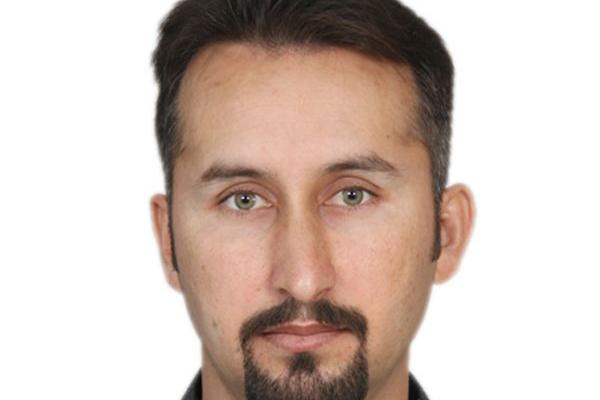Event date:
Sep 20 2021 2:30 pm

## Numerical Solutions of Some Nonlinear Fractional Flow Problems

Supervisor
Dr. Amer Rasheed
Student
Abdul Quayam Khan
Venue
Zoom Meetings (Online)
Event
PhD Thesis defense

Final Defense Committee:

• Dr. Imran Naeem (Convener, Mathematics - LUMS)

• Dr. Naveed ul Hassan (EE – LUMS, Examiner)

• Dr. Sultan Sial (Mathematics – LUMS, Examiner)

• Dr. Amer Rasheed (Mathematics – LUMS, Advisor)

Abstract:

Physical models of viscoelastic flow in terms of fractional order derivatives is a fascinating subject, in various fields of engineering, fluid dynamics, quantum computing, etc. The viscoelastic fluid models represent more realistic behavior as compared to the integer order derivatives in fluid dynamics. This is mainly because of the hereditary effects are taken into account by the fractional order models while formulating the flow problems. Mathematical models for such phenomenon usually result in the form of fractional partial differential equations (PDEs). In order to solve the fractional order PDEs, there are several analytical methods such as Laplace transform and Fourier transform methods in literature which had been usually employed to solve linear fractional order PDEs. However, it is difficult to solve non-linear fractional order PDEs using these analytical techniques. Hence, the researchers use numerical techniques to find approximate solutions for such fractional PDEs.

In this thesis, we have highlighted the numerical techniques alongwith the error and stability analysis of the schemes have been presented. Numerical methods, such as finite element and finite difference methods, along with L1 algorithm approximation, have been employed to investigate the approximate solutions. The simulations of flow fields as well as pertinent physical parameters and fractional order parameter effects on the fluid flow of some non-linear viscoelastic fluid problems have been discussed. A code in MATHLAB has been developed for simulations of the underlying models. Finally, the graphical illustration along with the physical interpretations have been presented for each problem. Conclusions and future directions are discussed at the end of thesis.

Join Zoom Meeting

Meeting ID: 935 4339 2760